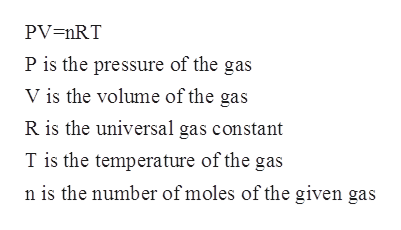# Hydrogen and Nitrogen gas react to form ammonia according to the following equation:3H2 (g) + N2 (g) --> 2NH3 (g)2.0L of hydrogen gas at a pressure of 1.10atm and a temperature of 55 degrees Celsius are reacted with 3.0L of nitrogen gas at a pressure of 0.90atm and a temperature of 55 degrees Celsius. How many grams of ammonia are produced?

Question
178 views

Hydrogen and Nitrogen gas react to form ammonia according to the following equation:

3H2 (g) + N2 (g) --> 2NH3 (g)

2.0L of hydrogen gas at a pressure of 1.10atm and a temperature of 55 degrees Celsius are reacted with 3.0L of nitrogen gas at a pressure of 0.90atm and a temperature of 55 degrees Celsius. How many grams of ammonia are produced?

check_circle

Step 1

The number of moles for a given chemical species refers to the ratio of mass of the species to that of its molar mass. The formula is shown below:

Step 2

The ideal gas equation is known as the general gas equation and is a equation of state for a given hypothetical gas. The equation is given by:help_outlineImage TranscriptionclosePV=nRT P is the pressure of the gas V is the volume of the gas R is the universal gas constant T is the temperature of the gas n is the number of moles of the given gas fullscreen
Step 3

The given reaction i...

### Want to see the full answer?

See Solution

#### Want to see this answer and more?

Solutions are written by subject experts who are available 24/7. Questions are typically answered within 1 hour.*

See Solution
*Response times may vary by subject and question.
Tagged in

### Physical Chemistry